# Follow-Up to "Pressure Profile"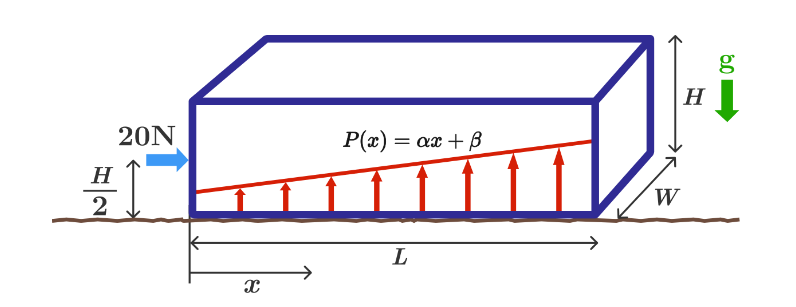I posted a problem called Pressure Profile a few days ago. When I wrote the problem, I assumed that when you push on a box without causing it to slide (there is friction), the normal reaction pressure varies linearly with the position along the box.

And sure enough, you can uniquely solve for a linear pressure profile which maintains the box in stasis. This is done by writing and solving two equations which describe the conditions for stasis (2 equations and 2 unknowns).

I then wondered what would happen if we assumed a quadratic pressure variation of the form $P(x) = \alpha x^{2} + \beta x + \gamma$. Now there are three unknown parameters, but still only two equations. I've found that if you simply choose the $\gamma$ parameter and solve for the other two, you can easily come up with a quadratic profile which has the following properties:

1) Satisfies the two equations for stasis
2) Results in positive upward pressure for all values of x between 0 and L

And there are presumably infinitely many such solutions, since I've already just casually found three quadratic profiles that have the above two properties. I suppose it would be possible to find solutions of even higher order as well (5th, 11th, 27th, etc.).

So my questions are these:

1) In the real world, would the pressure profile indeed be linear, as I originally assumed when posting the problem?

2) If so, how does nature know not to choose any higher-order variations in the pressure, since they appear to work, as far as I can tell?

3) Is there another constraint that forces a linear variation that I have simply not considered?

Appendix

For reference, here's the derivation for the general quadratic pressure profile, of which a linear profile is a special case.

$dA = W dx$ $P(x) = \alpha x^{2} + \beta x + \gamma$ $dF = P dA$ $d\tau = Px dA$

$Force: \frac{Mg}{W} = \int_{0}^{L} (\alpha x^{2} + \beta x + \gamma) dx \\ \implies \frac{Mg}{W} = \alpha \frac{L^3}{3} + \beta \frac{L^2}{2} + \gamma L$

$Torque \, about \, (0,0): \frac{Mg}{W} \frac{L}{2} + \frac{F}{W} \frac{H}{2} = \int_{0}^{L} (\alpha x^{3} + \beta x^{2} + \gamma x) dx \\ \implies \frac{Mg}{W} \frac{L}{2} + \frac{F}{W} \frac{H}{2} = \alpha \frac{L^4}{4} + \beta \frac{L^3}{3} + \gamma \frac{L^2}{2}$

By choosing the $\gamma$ parameter and solving the two equations for $\alpha$ and $\beta$, we can generate numerous quadratic solutions. By setting $\alpha$ to zero and solving for the other two, we can uniquely generate a linear profile. Here are three example profiles that seem to work (pressure is positive between 0 and L and the two equations for stasis are satisfied):

1) $\alpha = 0,\beta = 7.5,\gamma = 42.5$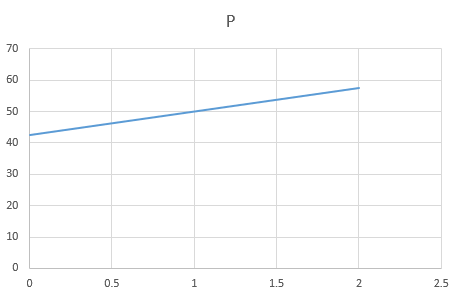2) $\alpha = 11.25,\beta = -15.0,\gamma = 50.0$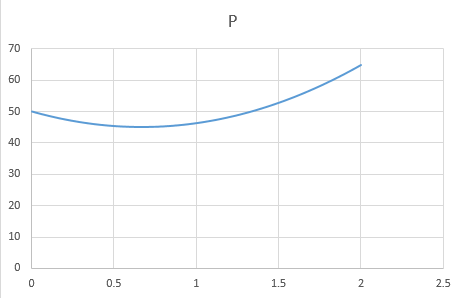3) $\alpha = -33.75,\beta = 75.0,\gamma = 20.0$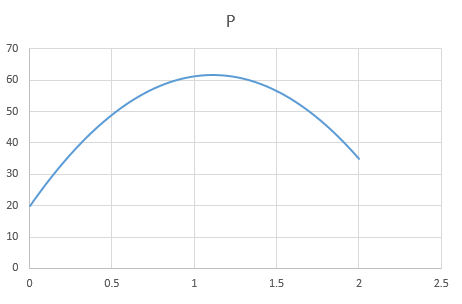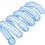Note by Steven Chase
3 years, 7 months ago

This discussion board is a place to discuss our Daily Challenges and the math and science related to those challenges. Explanations are more than just a solution — they should explain the steps and thinking strategies that you used to obtain the solution. Comments should further the discussion of math and science.

When posting on Brilliant:

• Use the emojis to react to an explanation, whether you're congratulating a job well done , or just really confused .
• Ask specific questions about the challenge or the steps in somebody's explanation. Well-posed questions can add a lot to the discussion, but posting "I don't understand!" doesn't help anyone.
• Try to contribute something new to the discussion, whether it is an extension, generalization or other idea related to the challenge.

MarkdownAppears as
*italics* or _italics_ italics
**bold** or __bold__ bold
- bulleted- list
• bulleted
• list
1. numbered2. list
1. numbered
2. list
Note: you must add a full line of space before and after lists for them to show up correctly
paragraph 1paragraph 2

paragraph 1

paragraph 2

[example link](https://brilliant.org)example link
> This is a quote
This is a quote
    # I indented these lines
# 4 spaces, and now they show
# up as a code block.

print "hello world"
# I indented these lines
# 4 spaces, and now they show
# up as a code block.

print "hello world"
MathAppears as
Remember to wrap math in $$ ... $$ or $ ... $ to ensure proper formatting.
2 \times 3 $2 \times 3$
2^{34} $2^{34}$
a_{i-1} $a_{i-1}$
\frac{2}{3} $\frac{2}{3}$
\sqrt{2} $\sqrt{2}$
\sum_{i=1}^3 $\sum_{i=1}^3$
\sin \theta $\sin \theta$
\boxed{123} $\boxed{123}$

Sort by:

Well, what forces are on a molecule in the fluid, and in particular at the surface?

Staff - 3 years, 7 months ago

Gravity acts at all points inside the box. An external force is applied at the left side of the box. There is an upward normal reaction from the surface upon which the box sits, which can be described in terms of a variable pressure. There is a horizontal friction force which keeps the box from sliding. And there should also be some internal horizontal forces within the box keeping the various differential box slices from moving horizontally. If there weren't, the friction forces would cause them to move.

- 3 years, 7 months ago

I see, originally I assumed you were talking about a liquid in an accelerating box—my mistake.

Are you assuming that the box doesn't deform at all under the force?

Staff - 3 years, 6 months ago

- 3 years, 6 months ago

It would be great if you could add your calculation for one or two of the profiles to the note, so we can really get at this.

Staff - 3 years, 6 months ago

yes , i think so too and when i solved the problem i had to assume that too , but i say you should do like i suggest here @Steven Chase at a distance x consider a strip or a should say a cuboid of length dx and the normal reaction force on it would be responsible for the weight balancing and if we consider it a normal block , the force applied at $\cfrac {H}{2}$ would vary linearly along it and when you balance torques you get the normal reaction and it is correct because here you get the real thing and you do not have o assume anything , what do you say @Steven Chase

- 3 years, 7 months ago

Greetings. I'm not sure I followed that. What additional constraint do you think forces a linear profile?

- 3 years, 7 months ago

the density of the cuboid is supposed to be linear ( i wrote a "normal block ") that's why i said the force applied varies linearly along the block ....if the density is some function of x , then it would not vary linearly , do you follow it now ? i would love if post a question on that when the density rises exponentially :) @Steven Chase

- 3 years, 7 months ago

Ok, I think I see what you mean. Yeah, feel free to post a follow-up problem if you want.

- 3 years, 7 months ago